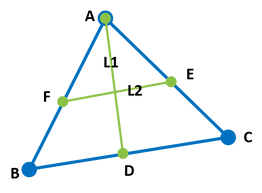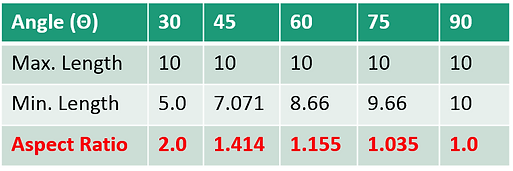top of page

## Introduction:

• The ratio between largest and smallest characteristic dimension of an element is known as the Aspect Ratio.

• Large aspect ratios increase the inaccuracy of the finite element representation and have a detrimental effect on convergence of Finite Element Solutions.

• An aspect ratio of 1 is ideal but cannot always be maintained. In general the aspect ratios are maintained in between 1 to 5 at critical areas in a domain where derivatives of field variable are significant.

• Along with solution accuracy the poor element shapes will often cause convergence problems in nonlinear analyses.

• Aspect ratio is one of the other mesh quality parameters like Skewness, Warping, Parallel deviation, Maximum corner angle, Jacobian and Orthogonal Quality.

• Maintaining element quality is always a challenge for analyst during mesh convergence studies. Though element size is minimized it is of no use if they are violating quality requirements.

• So, it is important to know how to calculate these parameters for different Element Shapes.

• This Presentation deals with how to calculate Aspect ratio for 2D elements.

## Example Mesh with Aspect Ratios (A.R):

###### Quad elements with Aspect Ratio: 1###### Ideal Shapes for Triangular and Quadrilateral Elements## 1. Aspect Ratio Calculation for Triangular Elements:

Finding The Length Ratio of Rectangle-1 Formed with L1 as Axis:

• From the triangle "ABC" join the mid point and opposite corner of each side (L1). Join two mid points of other adjacent sides (L2).

• Fixing L1 as axis, draw parallel lines to L1 at mid points of the adjacent sides at E & F.

• Draw perpendicular lines to L1 at A & D.

• Find the Length Ratio between the Max. Side to Mini. side of the Rectangle-1 Formed using L1 (The procedure is illustrated in the following figure).###### Triangular Element with L1 & L2###### Rectangle-1 formed with L1

Finding The Length Ratio of Rectangle-2 Formed with L2 as Axis:

• Similarly, Fixing L2 as axis, draw parallel lines to L2 at opposite corner and mid point of opposite side i.e. at A & D.

• Draw perpendicular lines to L2 at E & F.

• Find the Length Ratio between the Max. side to Mini. Side of the Rectangle-2 formed using L2 (The Procedure is illustrated in the following figure).###### Rectangle-2 formed with L2
• Repeat these steps for the three corners and construct all “6” Rectangles (formed with L3 & L4, L5 & L6) and find the length ratios.

• Find the Maximum Length Ratio out of the Six Rectangles formed.##### Aspect Ratio Calculation for Equilateral Triangle:

​Each Side of the Triangular Element : 50mm (AB=BC=CA)

Each corner angle : 60 degree (∠A=∠B=∠C=60 degree)###### Equilateral Triangle###### Construct rectangle with Ref. L1###### Construct rectangle with Ref. L2

Similarly, by constructing rectangles for all the other corners, the values of maximum and minimum side lengths will be same as measured above.## 2. Aspect Ratio Calculation for Quadrilateral Elements:

Finding The Length Ratio of Rectangle-1 formed with L1 as Axis:

• From the Quadrilateral "ABCD" join the mid points of opposite sides. These two lines form the axis lines L1 & L2.

• Fixing L1 as axis, draw parallel lines to L1 at mid points of the other opposite sides at E & G.

• Draw perpendicular lines to L1 at F & H.

• Find the Ratio between the Max. Side to Mini. side of the rectangle-1 formed using L1 (Procedure is illustrated in the following figure).###### Rectangle-1 formed with L1

Finding The Length Ratio of Rectangle-2 formed with L2 as Axis:

• Similarly, Fixing L2 as axis, draw parallel lines to L2 at mid points of the other opposite sides at F & H.

• Draw perpendicular lines to L2 at E & G.

• Find the ratio between the Max. side to Mini. Side of the Rectangle-2 formed using L2 (Procedure is illustrated in the following figure).###### Rectangle-2 formed with L2

So, The Aspect Ratio is Maximum of the two length ratios formed by L1 & L2.

##### Aspect Ratio Calculation for Square:

​Each Side of the Square Element : 50mm (AB=BC=CA)

Each corner angle : 90 degree (∠A=∠B=∠C=∠D=90 degree)###### Square Element with L1 & L2 Axis

Since, all sides are equal (AB=BC=CD=DA) and L1 is Perpendicular to L2, the rectangles constructed by fixing L1 & L2 as Axes are same as "ABCD". So, The aspect ratio is Maximum of the two length ratios formed by L1 & L2.##### Aspect Ratio Calculation for Rhombus:
• Rhombus is a quadrilateral all of whose sides have the same length (AB=BC=CD=DA).

• Assuming an element in the shape of a Rhombus with side length of 10mm each.###### Rhombus shaped Element with L1 & L2 Axis
• By following the procedure iterated for the Quadrilateral Element, construct rectangles about EF and GH as shown in below.

• Find the ratio of Max. to Min. Side lengths of the rectangles formed.###### Rectangle-1 formed with GH###### Rectangle-2 formed with GHParametric Study:

• By changing the angle Θ from 10 degree to 90 degree variation in the aspect ratio is studied and tabulated below.References:

• A First Course in the Finite Element Method, 4th Edition, by Logan, D. L. Boston (Page no: 351)

• Finite element procedures, Klaus-Jurgen Bathe, (Page no: 381)

• Fundamentals of Finite Element Analysis by Hutton DV, (Page no: 194)

• Finite Element Modeling for Stress Analysis - Robert D. Cook (page no: 110)

• Ansys, Inc. Theory Reference, Ansys Release 9.0, 2004 (Chapter: 13.7.3)

Note: * Ansys is the registered trademark of Ansys Inc.

Aspect Ratio Tria